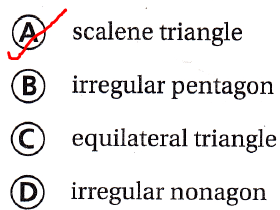Refer to our Texas Go Math Grade 5 Answer Key Pdf to score good marks in the exams. Test yourself by practicing the problems from Texas Go Math Grade 5 Module 11 Assessment Answer Key.

Vocabulary

Choose the best term from the box.

Question 1.
A closed plane figure with all sides congruent and all angles congruent is called a ____. (p.410)
We know that,
A closed plane figure with all sides congruent and all angles congruent is called a “Regular Polygon”
Hence, from the above,
We can conclude that
The term that is suitable for the given statement is: Regular Polygon

Question 2.
Line segments that have the same length or angles that have the same measure are ___. (p. 410)
We know that,
Line segments that have the same length or angles that have the same measure are “Congruent”
Hence, from the above,
We can conclude that
The term that is suitable for the given statement is: Congruent

Concepts and Skills

Draw the triangle in the correct group. TEKS 5.5.A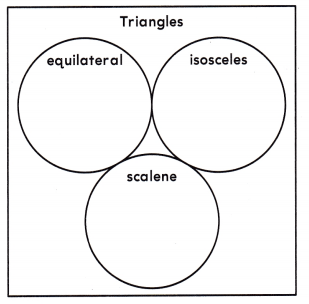Question 3.
All three sides are 2 cm long.
It is given that
All three sides are 2 cm long.
Now,
We know that,
An “Equilateral Triangle” has three congruent sides
Hence, from the above,
We can conclude that
The representation of the given triangle in the given Venn diagram is:Question 4.
Two sides are 2 cm long. The third side is 1 cm long.
It is given that
Two sides are 2 cm long. The third side is 1 cm long.
Now,
We know that,
An “Isosceles triangle” has 2 congruent sides
Hence, from the above,
We can conclude that
The representation of the given triangle in the given Venn diagram is: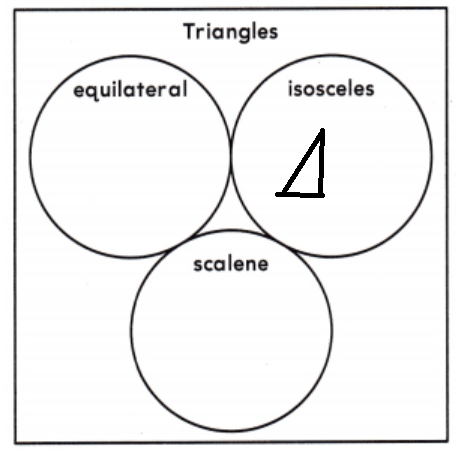Classify each triangle. Write isosceles, scalene, or equilateral. Then write acute, obtuse, or right. TEKS 5.5.A

Question 5.The given triangle is:Now,
From the given triangle,
We can observe that
All the sides are congruent and all the angle measures are less than 90°
Hence, from the above,
We can conclude that
The given triangle is an “Equilateral Triangle” and an “Acute Triangle”

Question 6.The given triangle is:Now,
From the given triangle,
We can observe that
Out of the three angles, two angle measures are the congruent
All the angle measures are not less than 90°
Hence, from the above,
We can conclude that
The given triangle is an “Isosceles Triangle” and an “Obtuse Triangle”

Classify the quadrilateral in as many ways as possible. Write quadrilateral, parallelogram, rectangle, rhombus, square, or trapezoid. TEKS 5.5.A

Question 7.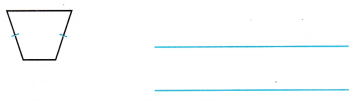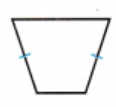Now,
We can observe that
There are only 1 pair of parallel sides
Hence, from the above,
We can conclude that
The given quadrilateral is: Trapezium (or) Trapezoid

Question 8.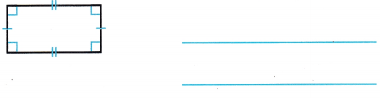Now,
We can observe that
The parallel sides are congruent
All the angle measures are 90°
Hence, from the above,
We can conclude that

Question 9.
What type of triangle is shown below? TEKS 5.5.A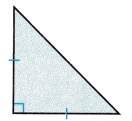(A) right isosceles
(B) right scalene
(C) equilateral
(D) obtuse scalene
The given triangle is:Now,
From the given triangle,
We can observe that
There is one perpendicular side and 2 congruent sides
Hence, from the above,
We can conclude that
The type of triangle that is given is: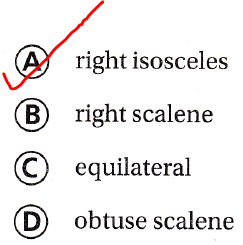Question 10.
Which of the following figures can never be classified as a square? TEKS 5.5.A
(A) rectangle
(B) parallelogram
(C) rhombus
(D) trapezoid
We know that,
A square is always Rhombus
A rhombus is sometimes a square
Hence, from the above,
We can conclude that
The figures that can never be classified as a square is: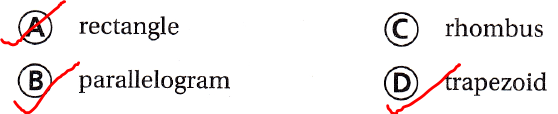Question 11.
Which of the following CANNOT be used for the unknown shape in the graphic organizer? TEKS 5.5.A(A) scalene triangle
(B) irregular pentagon
(C) equilateral triangle
(D) irregular nonagon